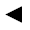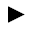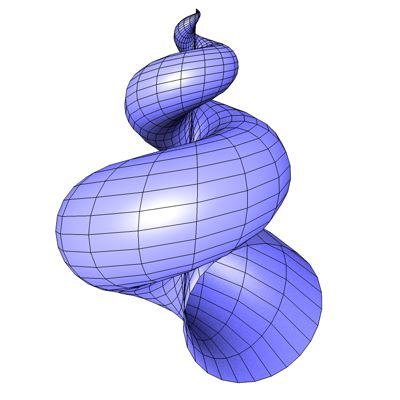﻿﻿﻿ math handbook calculator - Fractional Calculus Computer Algebra System software+++=﻿[back]

## 3.13. slug

[next]The worm [ 6 ] is represented by the following equations. To keep the formulas a little clearer, we use the constant h.

 h = e u/(6pi) 3-51
 x = a (1 - h) cos(u) cos(0.5 v) cos(0.5 v) 3-52 y = 1 - e u/(b pi) - sin(v) + h sin(v) 3-53 z = a (-1 + h) sin(u) cos(0.5 v) cos(0.5 v) 3-54

To represent the area, the two parameters u and v must have the following values ​​(definition range).

 u is an element from the set of numbers [0, 6 pi] v is an element of the number set [0, 2 pi]

The value u Max determines the number of windings of the worm, with u Max = 6 pi there are 3 windings.

The domain of definition of v is required to get a complete circle as a cross-section, it cannot be changed with the plugin.Fig. 17